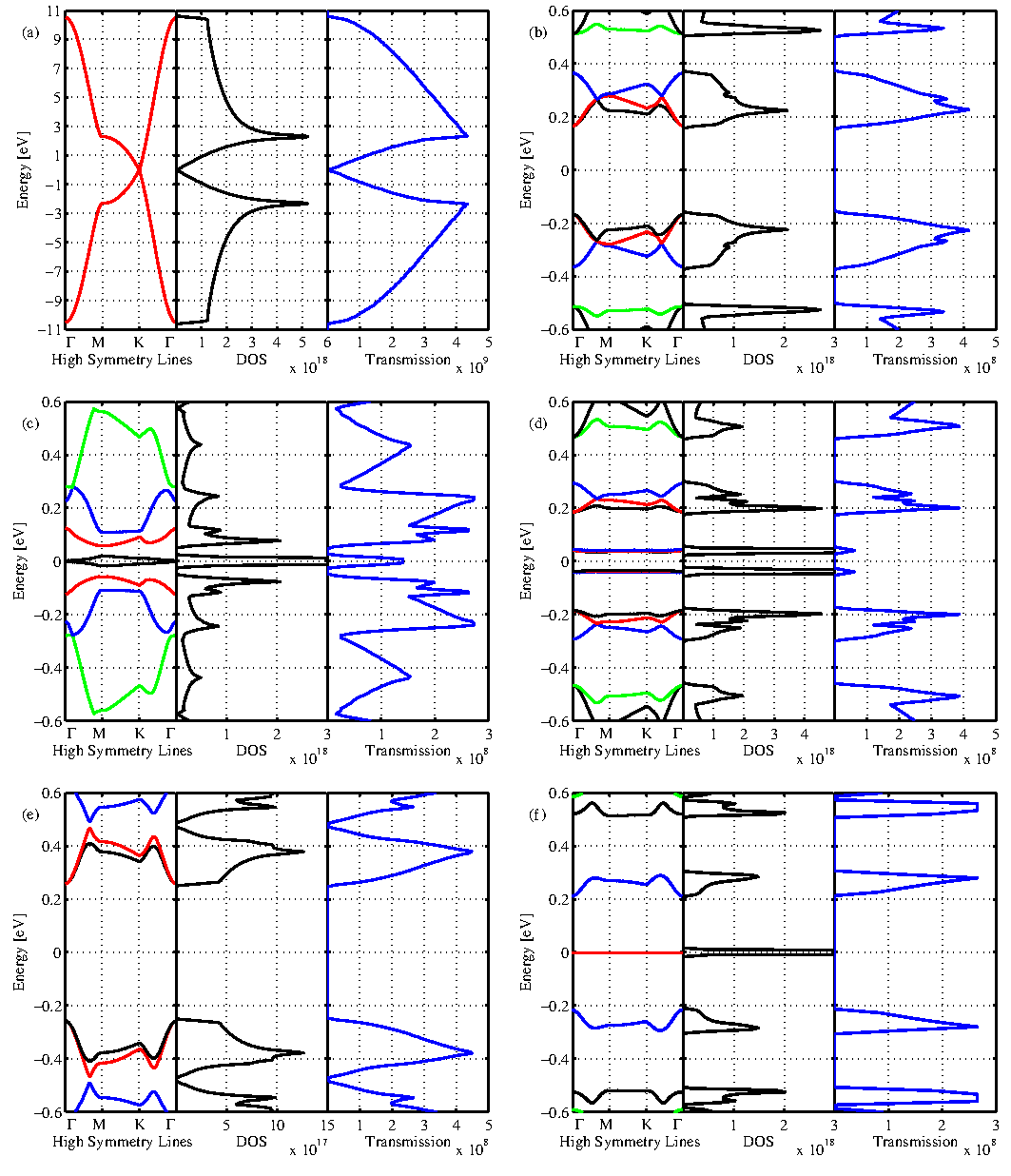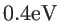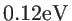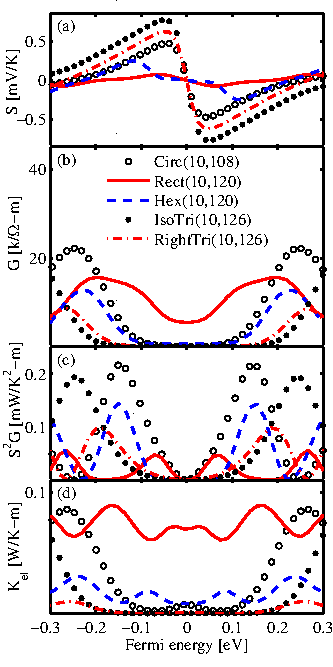Next: 3.2.3 Phononic Structure Up: 3.2 Thermoelectrics of Graphene Antidot Lattices Previous: 3.2.1 Geometrical Structure   Contents

## 3.2.2 Electronic StructureThe Seebeck coefficient and power factor are sensitive to the details of the density of states and the asymmetry between electrons and holes [85,86]. The electronic band structures of GALs are calculated using a third nearest-neighbor tight-binding method (Sec 2.1.1). By introducing the antidots in the graphene sheet, the zero band-gap graphene can be converted into a narrow band-gap semiconductor [26,27] (see Fig. 3.13). This issue plays an important role in thermoelectric applications. In contrast to pristine graphene, GALs have a beneficial band-gap, so that one can suppress either the electron or the hole current to obtain unipolar conduction. For example, by adjusting the Fermi level near the conduction band the hole current will be negligible. The electron-hole asymmetry with respect to the Fermi level depends on the band-gap, on the sharp features of transmission, on the width of the first conduction subband, and on the value of the transmission. At room temperature, the width of the thermal broadening function is about. Therefore, a band-gap aroundand a first conduction subband width larger thanwill be ideal for thermoelectric applications.

In RightTri(10,126), there are some localized midgap states, see Fig. 3.13-f, as a result of sublattice-symmetry breaking [84,87]. They have a zero group velocity and can not contribute to the carrier transport. Although RightTri(10,126) has the sharpest features in the transmission and its transport band-gap is about, the width of the first conduction subband of RightTri(10,126) is only. As a result, it has a high Seebeck coefficient and a low electrical conductance, see Fig. 3.14. The first conduction subband of a Rect(10,120) has a non-zero group velocity. Therefore, the rectangular GAL is considered as a zero band-gap material and as a result, the Seebeck coefficient will be small which is detrimental to thermoelectric applications. In a Hex(10,120), the first conduction and valence subbands are quasi-flat bands due to existence of some edge carbon atoms which have only one nearest neighbor . As shown in Fig. 3.13-d, these bands have a small group velocity and have a small contribution to electron transport. As a result, the maximum value of the Seebeck coefficient of Hex(10,120) is not very large and is located close to the band-edge of the second conduction subband. On the other hand, the electrical conductance peaks close to the second subband-edge. Therefore, Hex(10,120) has the third highest power factor among the GALs with different antidot shapes.On the other hand, the band-gap and the first conduction subband width of Circ(10,108) and IsoTri(10,126) are nearlyand, respectively. They also have the highest transmissions. Therefore, as shown in Fig. 3.14, they are the best thermoelectric GALs in terms of the power factor. Because of a sharp feature in the transmission, Circ(10,108) has the highest power factor of the GALs considered. In addition, as shown in Fig. 3.14-d the electron contribution to the thermal conductance can be neglected in comparison with the lattice thermal conductance (see Table 3.2).Next: 3.2.3 Phononic Structure Up: 3.2 Thermoelectrics of Graphene Antidot Lattices Previous: 3.2.1 Geometrical Structure   Contents
H. Karamitaheri: Thermal and Thermoelectric Properties of Nanostructures